# Fractions + natural numbers - math problems

#### Number of problems found: 48

• Reminder and quotientThere are given the number C = 281, D = 201. Find the highest natural number S so that the C:S, D:S are with the remainder of 1,
• Drawing from a hatWhen drawing numbers from a hat from 1 to 35, we select random given numbers. What is the probability that the drawn numbers will be divisible by 8 and 2?
• Ratio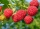Alena collected 7.8 kg of blueberries, 2.6 kg of blackberries, and 3.9 kg of cranberries. Express the ratio in the smallest natural numbers in this order.
• What is 11What is the quotient of Three-fifths and 1 Over 10?
• Balls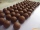From the bag with numbered balls (numbers 1,2,3,. ..20) we pick one ball. What is the probability of choosing a number containing 1?
• Diamonds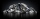If simva had 8 diamond and cuts them each into 1/3 pieces, then how many pieces does she have?
• Unknown numberIf I reduced the sum of the numbers 70 and the unknown number three times, I would get 100. what is the unknown number?
• FractionFraction ? write as fraction a/b, a, b is integers numerator/denominator.
• AddingDivide number 135 into two additions so that one adds 30 more than 2/5 of the other add. Write the bigger one.
• Pupils 7There are 40 pupils in a certain class. 3/5 of the class are boys. How many are girls?
• The difference 2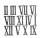The difference between the two numbers is 25. The smaller number is 1/6th of the larger number. What is the value of the smaller number?
• NumbersDetermine the number of all positive integers less than 4183444 if each is divisible by 29, 7, 17. What is its sum?
• Reminder and quotientThere are given numbers A = 135, B = 315. Find the smallest natural number R greater than 1 so that the proportions R:A, R:B are with the remainder 1.
• How manyHow many integers are greater than 547/3 and less than 931/4?
• FortyForty five of the 80 students were girls. What is the ratio of girls to boys?
• Say we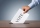Say we have 70 voting delegates and we need a 2/3 vote What would that number be? Also same as above but in this virtual world, it converts to percentages. What would the % number of positive votes be?
• Candies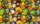There are 150 candies in the bowl. Ivan took 7 thirtieths, Charles 6 fifteenths, and Thomas 3 tenths. How many sweets are left in the bowl?
• Prime divisorsFind 2/3 of the sum's ratio and the product of all prime divisors of the number 120.
• Fraction to decimal infinite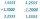Determine which digit is at 1000th place after the decimal point in the decimal expansion of the fraction 9/28.
• Homework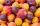In the crate are 18 plums, 27 apricot and 36 nuts. How many pieces of fruit left in the crate when Peter took 8 ninth: 1. nuts 2. apricots 3. fruit 4. drupe

Do you have an interesting mathematical word problem that you can't solve it? Submit a math problem, and we can try to solve it.

We will send a solution to your e-mail address. Solved examples are also published here. Please enter the e-mail correctly and check whether you don't have a full mailbox.

Please do not submit problems from current active competitions such as Mathematical Olympiad, correspondence seminars etc...

Need help to calculate sum, simplify or multiply fractions? Try our fraction calculator. Fraction Word Problems. Natural numbers - math problems.